facility-location

If any part of this notebook is used in your research, please cite with the reference found in README.md.

## Demostrating network-based optimal facility location modeling

### Integrating pysal/spaghetti with Cbc and ortools

Author: James D. Gaboardi jgaboardi@gmail.com

This notebook provides a use case for:

1. Introducing four fundamental optimal facility location models
2. Utilizing the data structures provided by spaghetti as input for optimization problems
3. Solving the models, and comparing and visualizing the results
%load_ext watermark
%watermark

2020-01-20T23:07:33-05:00

CPython 3.7.3
IPython 7.10.2

compiler   : Clang 9.0.0 (tags/RELEASE_900/final)
system     : Darwin
release    : 19.2.0
machine    : x86_64
processor  : i386
CPU cores  : 4
interpreter: 64bit


In addtion to the base spaghetti requirements (and their dependecies), this notebook requires installations of:

• descartes
• $conda install -c conda-forge descartes • geopandas • $ conda install -c conda-forge geopandas
• matplotlib
• $conda install matplotlib • ortools • $ pip install ortools
• seaborn
• $conda install seaborn import spaghetti import sys, warnings from collections import OrderedDict import geopandas import libpysal from libpysal import cg, examples import matplotlib import matplotlib.pyplot as plt import matplotlib.lines as mlines import matplotlib.patches as mpatches from matplotlib.colors import ListedColormap import numpy import ortools from ortools.linear_solver import pywraplp import seaborn import shapely from shapely.geometry import Point try: from IPython.display import Markdown as md from IPython.display import set_matplotlib_formats set_matplotlib_formats("retina") except ImportError: pass %matplotlib inline %watermark -w %watermark -iv  watermark 2.0.2 spaghetti 1.4.0 libpysal 4.2.0 matplotlib 3.1.2 ortools 7.4.7247 geopandas 0.6.2 numpy 1.17.3 shapely 1.6.4.post2 seaborn 0.9.0  ### 1.1 Scenario: The Neighborhood X Planning Committee has been asked by residents to set up a Little Free Library program to supplement the community's growing reading needs, as 15 families just moved in bring the total household count to 400. Since the neighborhood can't afford to have a library on every corner, the committee must decide on where to locate them and how to do so in a transparent manner. There are currently 14 candidate sites in the neighborhood for Little Free Libraries and the community can afford to support up to 5 locations, but would preferably support 3 locations with the extra budget going towards new books and unforseen maintenance costs. The committee also must locate all the libraries within 1 km of all households, but preferably with .8km. As it turns out one of the committee members is an Operations Research professor at the local university, Dr. Minimax, and volunteers to build 4 models to optimally site the Little Free Libraries acording to objective functions and specific sets of contraints. She puts forth 4 facility location models: • Location Set Covering Problem • Site the minimum number of facilities to cover all demand (clients) within a specified service radius. • Originally Published: Toregas, C. and ReVelle, Charles. 1972. Optimal Location Under Time or Distance Constraints. Papers of the Regional Science Association. 28(1):133 - 144. • p-median Problem • Site a predetermined number of facilities while minimizing total weighted distance. • Originally Published: S. L. Hakimi. 1964. Optimum Locations of Switching Centers and the Absolute Centers and Medians of a Graph. Operations Research. 12 (3):450-459. • p-center Problem • Site a predetermined number of facilities while minimizing the worst-case travel distance. • Originally Published: S. L. Hakimi. 1964. Optimum Locations of Switching Centers and the Absolute Centers and Medians of a Graph. Operations Research. 12 (3):450-459. • Maximal Covering Location Problem • Site a predetermined number of facilities while maximizing demand coverage within a specified service radius. • Originally Published: Church, R. L and C. ReVelle. 1974. The Maximal Covering Location Problem. Papers of the Regional Science Association. 32:101-18. ### 1.2 Set parameters as per the scenario layed out above # n clients and n facilities client_count, facility_count = 400, 14 # candidate facilites to site p_facilities = 3 # maximum coverage meters max_coverage = 1000.0 # minimum coverage meters min_coverage = 800.0 random_seeds = {"client": 3006, "facility": 1520} title = "Neighborhood X"  ### 1.3. Define a class and functions for solving the models and analyzing the results class FacilityLocationModel: """Solve a facility location optimization model Parameters ---------- name : str Problem model name; must also be defined as a class method. cij : numpy.ndarray cost matrix from origins (index of i) to destination (index of j). Default is None. ai : numpy.ndarray Client weight vector. Default is None. s : float service radius. Default is None. p : int Density of facilities to site. Default is None. write_lp : str file name (and path) of the LP file to write out. print_sol : bool print select results. Default is True. Methods ------- build_lscp : build location set covering problem build_pmp : build p-median problem build_pcp : build p-center problem build_mclp : build maximal covering location problem add_vars : add variables to a model add_constrs : add contraints to a model add_obj : add an objective function to a model optimize : solve a model record_decisions : record optimal decision variables non_obj_vals : record non-objective values stats (eg. percent covered) print_results : print selected results Attributes ---------- model : ortools.linear_solver.pywraplp.Solver proxy of <Swig Object of type 'operations_research::MPSolver *' n_cli : int total client sites r_cli : range iterable of client sites n_fac : int total candidate facility sites r_fac : range iterable of candidate facility sites aij : numpy.ndarray binary coverage matrix from cij (within s service radius) sij : numpy.ndarray demand weighted cost matrix as (ai * cij). fac_vars : dict facility decision variables cli_vars : dict client decision variables W : ortools.linear_solver.pywraplp.Variable minimized maximum variable in the p-center problem formulation lp_formulation : str linear programming formulation of the model solve_minutes : float solve time in minutes obj_val : int or float model objective value fac2cli : dict facility to client relationship lookup cli2fac : dict client to facility relationship lookup fac2iloc : dict facility to dataframe index location lookup n_cli_uncov : int count of client location outside the service radius cli2ncov : dict client to covered by count lookup ncov2ncli : dict covered by count to client count lookup mean_dist : mean distance per person to the assigned facility perc_served : percentage of weighted clients covered in s """ def __init__( self, name, ai=None, cij=None, s=None, p=None, write_lp=None, print_sol=True ): # Set model information self.name = name # create a solver instance solver_instance = pywraplp.Solver.CBC_MIXED_INTEGER_PROGRAMMING # instantiate a model self.model = pywraplp.Solver(self.name, solver_instance) # Set parameters and indices # facility parameter if p: self.p = p # client count and range self.cij = cij self.n_cli = cij.shape self.r_cli = range(self.n_cli) # facility count and range self.n_fac = self.cij.shape self.r_fac = range(self.n_fac) # demand parameter if ai is not None: self.ai = ai self.ai_sum = ai.sum() # weighted demand try: self.sij = self.ai * self.cij except ValueError: self.ai = self.ai.values.reshape(self.n_cli, 1) self.sij = self.ai * self.cij # if the model has a service radius parameter if s: self.s = s # binary coverage matrix from cij self.aij = numpy.zeros(self.cij.shape) self.aij[self.cij <= self.s] = 1.0 # Set decision variables, constraints, and objective function try: getattr(self, "build_" + self.name)() except: raise AttributeError(self.name, "not a defined location model.") # solve self.optimize(write_lp=write_lp) # records seleted decision variables self.record_decisions() # record non-objective values stats (eg. percent covered) self.non_obj_vals() # print results if print_sol: self.print_results() def build_lscp(self): """ Integer programming formulation of the Location Set Covering Problem. Originally Published: Toregas, C. and ReVelle, Charles. 1972. Optimal Location Under Time or Distance Constraints. Papers of the Regional Science Association. 28(1):133 - 144. """ # Decision Variables self.add_vars() # Constraints self.add_constrs(constr=1) # set coverage constraints # Objective Function self.add_obj() def build_pmp(self): """Integer programming formulation of the p-median Problem. Originally Published: S. L. Hakimi. 1964. Optimum Locations of Switching Centers and the Absolute Centers and Medians of a Graph. Operations Research. 12 (3):450-459. Adapted from: -1- ReVelle, C.S. and Swain, R.W. 1970. Central facilities location. Geographical Analysis. 2(1), 30-42. -2- Toregas, C., Swain, R., ReVelle, C., Bergman, L. 1971. The Location of Emergency Service Facilities. Operations Research. 19 (6), 1363-1373. - 3 - Daskin, M. (1995). Network and discrete location: Models, algorithms, and applications. New York: John Wiley and Sons, Inc. """ # Decision Variables self.add_vars() # Constraints self.add_constrs(constr=2) # assignment constraints self.add_constrs(constr=3) # facility constraint self.add_constrs(constr=4) # opening constraints # Objective Function self.add_obj() def build_pcp(self): """Integer programming formulation of the p-center Problem. Originally Published: S. L. Hakimi. 1964. Optimum Locations of Switching Centers and the Absolute Centers and Medians of a Graph. Operations Research. 12 (3):450-459. Adapted from: Daskin, M. (1995). Network and discrete location: Models, algorithms, and applications. New York: John Wiley and Sons, Inc. """ # Decision Variables self.add_vars() # Constraints self.add_constrs(constr=2) # assignment constraints self.add_constrs(constr=3) # facility constraint self.add_constrs(constr=4) # opening constraints self.add_constrs(constr=5) # minimized maximum constraints # Objective Function self.add_obj() def build_mclp(self): """Integer programming formulation of the Maximal Covering Location Problem. Originally Published: Church, R. L and C. ReVelle. 1974. The Maximal Covering Location Problem. Papers of the Regional Science Association. 32:101-18. """ # Decision Variables self.add_vars() # Constraints self.add_constrs(constr=3) # facility constraint self.add_constrs(constr=6) # maximal coverage constraints # Objective Function self.add_obj() def add_vars(self): """Add variables to a model. """ # facility decision variables self.fac_vars = {j: self.model.IntVar(0, 1, "y[%i]" % (j)) for j in self.r_fac} # client decision variables if self.name == "mclp": self.cli_vars = { (i): self.model.IntVar(0, 1, "x[%i]" % (i)) for i in self.r_cli } if self.name == "pmp" or self.name == "pcp": self.cli_vars = { (i, j): self.model.IntVar(0, 1, "x[%i,%i]" % (i, j)) for i in self.r_cli for j in self.r_fac } # minimized maximum variable if self.name == "pcp": self.W = self.model.NumVar(0, self.model.infinity(), "W") def add_constrs(self, constr=None): """ Add constraints to a model. (1) set coverage constraints y1 + x2 >= 1 x1 + x3 >= 1 x2 >= 1 (2) assignment constraints x1_1 + x1_2 + x1_3 = 1 (3) facility constraints y1 + y2 + y3 = p (4) opening constraints - x1_1 + y1 >= 0 - x2_1 + y1 >= 0 - x3_1 + y1 >= 0 (5) minimax constraints cost1_1*x1_1 + cost1_2*x1_2 + cost1_3*x1_3 - W <= 0 (6) maximal coverage constraints - x1 + y1 + y3 >= 0 - x2 + y4 >= 0 Parameters ---------- constr : int {1, 2, 3, 4, 5, 6} Contraint type to add to model. See above for explanation. Default is None. """ # 1 - set covering constraints if constr == 1: for i in self.r_cli: self.model.Add( self.model.Sum( [self.aij[i, j] * self.fac_vars[j] for j in self.r_fac] ) >= 1 ) # 2 - assignment constraints elif constr == 2: for i in self.r_cli: self.model.Add( self.model.Sum([self.cli_vars[i, j] for j in self.r_fac]) == 1 ) # 3 - facility constraint elif constr == 3: self.model.Add( self.model.Sum([self.fac_vars[j] for j in self.r_fac]) == self.p ) # 4 - opening constraints elif constr == 4: for i in self.r_cli: for j in self.r_fac: self.model.Add(self.fac_vars[j] - self.cli_vars[i, j] >= 0) # 5 - minimax constraints elif constr == 5: for i in self.r_cli: self.model.Add( self.model.Sum( [self.cij[i, j] * self.cli_vars[i, j] for j in self.r_fac] ) <= self.W ) # 6 - max coverage constraints elif constr == 6: for i in self.r_cli: self.model.Add( self.model.Sum( [self.aij[i, j] * self.fac_vars[j] for j in self.r_fac] ) >= self.cli_vars[i] ) def add_obj(self): """ Add an objective function to a model. """ if self.name == "lscp": self.model.Minimize(self.model.Sum([self.fac_vars[j] for j in self.r_fac])) elif self.name == "pmp": obj = [ self.sij[i, j] * self.cli_vars[i, j] for i in self.r_cli for j in self.r_fac ] self.model.Minimize(self.model.Sum(obj)) elif self.name == "pcp": self.model.Minimize(self.W) elif self.name == "mclp": obj = [self.ai.flatten()[i] * self.cli_vars[i] for i in self.r_cli] self.model.Maximize(self.model.Sum(obj)) def optimize(self, write_lp=False): """ Solve the model. Parameters ---------- write_lp : bool write out the linear programming formulation """ def _redirect_to_file(self, text): """ Write out the model in linear programming format. Parameters ---------- text : str full lp formulation in str format """ original = sys.stdout sys.stdout = open(self.name + ".lp", "w") print(text) sys.stdout = original self.model.Solve() # linear programming formulation if write_lp: self.lp_formulation = self.model.ExportModelAsLpFormat(True) self._redirect_to_file(self.lp_formulation) # WallTime() in milliseconds self.solve_minutes = self.model.WallTime() * 1.66667e-5 self.obj_val = self.model.Objective().Value() def record_decisions(self): """record decision variable relationship folowing optimization. """ # facility-to-dataframe index location lookup self.fac2iloc = {v.name(): k for k, v in self.fac_vars.items()} # client-to-dataframe index location lookup self.cli2iloc = {} # facility-to-client lookup self.fac2cli = {} # record client/service relationships for j in self.r_fac: if self.fac_vars[j].solution_value() > 0: jvar = self.fac_vars[j].name() self.fac2cli[jvar] = [] for i in self.r_cli: ivar = None if self.name == "lscp": if self.aij[i, j] > 0: ivar = "x[%i]" % i self.fac2cli[jvar].append(ivar) elif self.name == "mclp": if self.cli_vars[i].solution_value() > 0: if self.aij[i, j] > 0: ivar = self.cli_vars[i].name() self.fac2cli[jvar].append(ivar) else: if self.cli_vars[i, j].solution_value() > 0: ivar = self.cli_vars[i, j].name() ivar = ivar.split(",") + "]" self.fac2cli[jvar].append(ivar) if ivar: self.cli2iloc[ivar] = i # client-to-facility lookup self.cli2fac = {} for cv in list(self.cli2iloc.keys()): self.cli2fac[cv] = [] for k, v in self.fac2cli.items(): if cv in v: self.cli2fac[cv].append(k) # count of uncovered clients self.n_cli_uncov = self.n_cli - len(self.cli2iloc.keys()) # clients of clients covered by n facilities if self.name == "lscp" or self.name == "mclp": self.cli2ncov = {} for c, fs in self.cli2fac.items(): self.cli2ncov[c] = len(fs) most_coverage = max(self.cli2ncov.values()) self.ncov2ncli = {} for cov_count in range(most_coverage + 1): if cov_count == 0: self.ncov2ncli[cov_count] = self.n_cli_uncov continue if not cov_count in list(self.cli2ncov.keys()): self.ncov2ncli[cov_count] = 0 for c, ncov in self.cli2ncov.items(): if ncov >= cov_count: self.ncov2ncli[cov_count] += 1 def non_obj_vals(self): """ """ if self.name == "pmp": self.mean_dist = self.obj_val / float(self.ai_sum) if self.name == "mclp": self.perc_served = (self.obj_val / float(self.ai_sum)) * 100.0 def print_results(self): """print select results """ print("Solve Time:", self.solve_minutes, "minutes") # solve time and objective value if self.name == "lscp": u1 = "facilities needed for total coverage within a " u2 = "%f meter service radius" % self.s if self.name == "pmp": u1 = "total weighted distance with " u2 = "%i selected facilities" % self.p if self.name == "pcp": u1 = "worst case distance with " u2 = "%i selected facilities" % self.p if self.name == "mclp": u1 = "residents within %f meters of " % self.s u2 = "%i selected facilities" % self.p units = u1 + u2 print("Obj. Value:", self.obj_val, units) if self.name == "pmp": print("Mean weighted distance per", "person: %f" % self.mean_dist) if self.name == "mclp": print( "Percent of %i" % self.ai_sum, "clients covered: %f" % self.perc_served ) # coverage values if self.name == "lscp" or self.name == "mclp": for ncov, ncli in self.ncov2ncli.items(): if ncov == 0: print("--- %i clients are not covered" % ncli) else: if ncov == 1: sp = "y" else: sp = "ies" print( "--- %i clients are covered" % ncli, "by %i" % ncov, "facilit" + sp, )  def add_results(model, cli_df, fac_df, print_solution=False): """Add decision variable relationships to a dataframe. Parameters ---------- model : ortools.linear_solver.pywraplp.Solver proxy of <Swig Object of type 'operations_research::MPSolver *' cli_df : geopandas.GeoDataFrame GeoDataFrame of client locations fac_df : geopandas.GeoDataFrame GeoDataFrame of facility locations print_solution : bool print out solution decision variables. Default is False. Returns ------- cli_df : geopandas.GeoDataFrame updated client locations fac_df : geopandas.GeoDataFrame updated facility locations """ col_name = model.name + "_sol" fillers = [[cli_df, "cli2fac"], [fac_df, "fac2cli"]] for df, attr in fillers: df[col_name] = df["dv"].map(getattr(model, attr)) df[col_name].fillna("closed", inplace=True) if print_solution: selected = fac_df[fac_df[col_name] != "closed"] for idx in selected.index: print("") print(selected.loc[idx, "dv"], "serving:", selected.loc[idx, col_name]) return cli_df, fac_df  def plotter( fig=None, base=None, plot_aux=None, buffered=None, model=None, pt1_size=None, pt2_size=None, plot_res=None, save_fig=False, title=None, figsize=(10, 10), ): """ Top-level scenario plotter for location analytics. Parameters ---------- fig : matplotlib.figure.Figure complete figure to plot. Default is None. base : matplotlib.axes._subplots.AxesSubplot individual axis to plot. Default is None. plot_aux : dict model data parameters dataframes to plot keyed by descriptive names. Default is None. plot_res : dict model data results dataframes to plot keyed by descriptive names. Default is None. buffered : see buffer distance from roads segments in plot_base. Default is None. pt1_size : float or float size of points to plot. pt1_size should always be the larger between pt2_size and pt1_size. Default is None. pt2_size : float or float size of points to plot. Default is None. model : ortools.linear_solver.pywraplp.Solver proxy of <Swig Object of type 'operations_research::MPSolver *' title : str plot title. Default is None. figsize : tuple Figure size for plot. Default is (12,12). save_fig : bool Default is False. Returns ------- add_to_legend : list items to add to legend """ for_multiplot = True if not fig and not base: for_multiplot = False fig, base = plt.subplots(1, 1, figsize=figsize) # add title if not for_multiplot: if model: title += " - " + model.name base.set_title(title, size=20) else: base.set_title(model.name, size=20) # plot non-results data if plot_aux: for k, df in plot_aux.items(): if k == "streets": df.plot(ax=base, lw=2, edgecolor="k", zorder=1) if k == "buffer": df.plot(ax=base, facecolor="y", lw=0.25, alpha=0.25, zorder=1) if k == "cli_tru": if plot_res: df = df[df[model.name + "_sol"] == "closed"] psize = pt2_size / 6.0 pcolor = "k" else: n_cli = df.shape psize = pt1_size pcolor = "r" df.plot(ax=base, markersize=psize, edgecolor="k", facecolor=pcolor) if k == "fac_tru": if plot_res: df = df[df[model.name + "_sol"] == "closed"] psize = pt2_size pcolor = "k" pmarker = "*" else: n_cli = df.shape psize = pt1_size pcolor = "b" pmarker = "o" df.plot( ax=base, markersize=psize, edgecolor="k", facecolor=pcolor, marker=pmarker, ) n_fac = df.shape if k == "cli_snp": df.plot( ax=base, markersize=pt2_size, edgecolor="k", facecolor="r", alpha=0.75, ) if k == "fac_snp": df.plot( ax=base, markersize=pt2_size, edgecolor="k", facecolor="b", alpha=0.75, ) add_to_legend = list(plot_aux.keys()) else: add_to_legend = None # plot results data if plot_res: dv_colors = dv_colorset(plot_res["fac_var"].dv) # facilities df = plot_res["fac_var"][plot_res["fac_var"][model.name + "_sol"] != "closed"] alpha = 1.0 / float(len(df.dv) - 2) if alpha > 0.5: alpha = 0.5 # decision variable info for legend dvs_to_leg = {} # plot facilities for dv in df.dv: fac = df[df.dv == dv] fac.plot( ax=base, marker="*", markersize=pt1_size * 3.0, alpha=0.8, zorder=3, edgecolor="k", facecolor=dv_colors[dv], ) # update decision variable info with set color dvs_to_leg[dv] = {"facecolor": dv_colors[dv]} # plot clients & service areas for f, c in model.fac2cli.items(): fc = plot_res["cli_var"][plot_res["cli_var"].dv.isin(c)] fc.plot( ax=base, markersize=50, edgecolor="k", facecolor=dv_colors[f], alpha=alpha, zorder=2, ) # update decision variable info with set client counts dvs_to_leg[f].update({"clients": fc.shape}) # create service area polygon service_area = concave_hull(df, fc, f) service_area.plot( ax=base, edgecolor="k", alpha=0.2, facecolor=dv_colors[f], zorder=1 ) else: dvs_to_leg = None if not model: class _ShellModel: """object to mimic model when not present """ def __init__(self, plot_aux): try: self.n_cli = plot_aux["cli_tru"].shape try: self.n_fac = plot_aux["fac_tru"].shape except KeyError: pass except KeyError: pass try: model = _ShellModel(plot_aux) except (TypeError, KeyError): model = None if not for_multiplot: # create legend patches patches = create_patches( model=model, for_multiplot=for_multiplot, pt1_size=pt1_size, pt2_size=pt2_size, buffered=buffered, legend_aux=add_to_legend, dvs_to_leg=dvs_to_leg, ) add_legend(patches, for_multiplot=for_multiplot) add_north_arrow(base) add_scale(base) if save_fig: plt.savefig(model.name + ".png") # if for a multiplot explicityly return items to add to legend if for_multiplot: return add_to_legend  def multi_plotter( models, plot_aux=None, plot_res=None, select=None, title=None, figsize=(14, 14), shape=(2, 2), ): """plot multiple base axes as one figure Parameters ---------- models : list solved model objects select : dict facility-to-selection count lookup. shape : tuple dimension for subplot array. Default is (2,2).s plot_aux : see plotter() plot_res : see plotter() title : see plotter() figsize : see plotter() """ pt1_size, pt2_size = 300, 60 # convert list of models to array mdls = numpy.array(models).reshape(shape) fig, axarr = plt.subplots( mdls.shape, mdls.shape, figsize=figsize, sharex="col", sharey="row" ) # add super title to subplot array plt.suptitle(title, fontsize=30) fig.subplots_adjust(hspace=0.1, wspace=0.005, top=0.925) # create each subplot for i in range(mdls.shape): for j in range(mdls.shape): add_to_legend = plotter( base=axarr[i, j], plot_aux=plot_aux, plot_res=plot_res, model=mdls[i, j], pt1_size=pt1_size, pt2_size=pt2_size, ) axarr[i, j].set_aspect("equal") add_to_legend = set(add_to_legend) # decision variable color set dv_colors = dv_colorset(plot_res["fac_var"].dv) dvs_to_leg = {f: dv_colors[f] for m in models for f in m.fac2cli.keys()} # set ordered dict of {iloc:fac_var, color, x-selected} # *** models can be any of the solved models dvs_to_leg = { models.fac2iloc[k]: (k, v, select[k]) for k, v in dvs_to_leg.items() } dvs_to_leg = OrderedDict(sorted(dvs_to_leg.items())) # create legend patches patches = create_patches( model=None, pt1_size=pt1_size, pt2_size=pt2_size, legend_aux=add_to_legend, dvs_to_leg=dvs_to_leg, for_multiplot=True, ) add_legend(patches, for_multiplot=True)  def add_north_arrow(base): """add a north arrow to an axes Parameters ---------- base : see plotter() """ arw = "rarrow, pad=0.25" bbox_props = dict(boxstyle=arw, fc="w", ec="k", lw=2, alpha=0.75) base.text( 221200, 267200, " z ", bbox=bbox_props, fontsize="large", fontweight="heavy", ha="center", va="center", rotation=90, )  def add_scale(base): """add a scale arrow to an axes Parameters ---------- base : see plotter() """ bbox_props = dict(boxstyle="round, pad=.5", fc="w", ec="0.5", alpha=0.7) base.text( base.get_xlim() + 75, base.get_ylim() + 75, "| ~.25km~ |", fontstyle="italic", bbox=bbox_props, )  def create_patches( model=None, pt1_size=None, pt2_size=None, buffered=None, legend_aux=None, dvs_to_leg=None, for_multiplot=False, ): """create all patches to add to the legend. Parameters ---------- for_multiplot : bool for a single plot (True), or multiplot (False). Default is False. model : see plotter() pt1_size : see plotter() pt2_size : see plotter() buffered : see plotter() legend_aux : see plotter() dvs_to_leg : see plotter() Returns ------- patches : list legend handles matching plotted items """ if pt1_size: ms1 = float(pt1_size) / 6.0 if pt2_size: ms2 = float(pt2_size) / 8.0 # empty legend spacing spacer = mpatches.Patch(edgecolor="None", facecolor="None", linewidth=0, label="") # all patches to add to legend patches = [] # streets -- always plot strs = mlines.Line2D([], [], color="k", linewidth=2, alpha=1, label="Streets") patches.extend([spacer, strs]) # non-results data if legend_aux: if "buffer" in legend_aux: label = "Street buffer (%sm)" % buffered strbuff = mpatches.Patch( edgecolor="None", facecolor="y", linewidth=2, alpha=0.5, label=label ) patches.extend([spacer, strbuff]) if "cli_tru" in legend_aux: try: if dvs_to_leg: pcolor = "k" msize = ms2 / 3.0 plabel = "Uncovered Households " + "($n$=%i)" % model.n_cli_uncov else: pcolor = "r" msize = ms1 plabel = "Households ($n$=%i)" % model.n_cli cli_tru = mlines.Line2D( [], [], color=pcolor, marker="o", ms=msize, linewidth=0, alpha=1, markeredgecolor="k", label=plabel, ) patches.extend([spacer, cli_tru]) except AttributeError: pass if "fac_tru" in legend_aux: if dvs_to_leg: pcolor = "k" msize = ms2 pmarker = "*" no_fac = model.n_fac - len(list(model.fac2cli.keys())) plabel = "Unselected Facilities ($n$=%i)" % no_fac else: pcolor = "b" msize = ms1 pmarker = "o" plabel = "Little Free Library candidates" + "($n$=%i)" % model.n_fac fac_tru = mlines.Line2D( [], [], color=pcolor, marker=pmarker, ms=msize, markeredgecolor="k", linewidth=0, alpha=1, label=plabel, ) patches.extend([spacer, fac_tru]) if "cli_snp" in legend_aux: label = "Households snapped to network" cli_snp = mlines.Line2D( [], [], color="r", marker="o", ms=ms2, linewidth=0, alpha=1, markeredgecolor="k", label=label, ) patches.extend([spacer, cli_snp]) if "fac_snp" in legend_aux: label = "LFL candidates snapped to network" fac_snp = mlines.Line2D( [], [], color="b", marker="o", ms=ms2, markeredgecolor="k", linewidth=0, alpha=1, label=label, ) patches.extend([spacer, fac_snp]) patches.extend([spacer]) # results data for single plot if dvs_to_leg and not for_multiplot: # add facility, client, and service area patches to legend for k, v in dvs_to_leg.items(): fdv_label = "Little Free Library %s" % k fdv = mlines.Line2D( [], [], color=v["facecolor"], marker="*", ms=ms1 / 2.0, markeredgecolor="k", linewidth=0, alpha=0.8, label=fdv_label, ) cdv_label = "Households served by %s " % k + "($n$=%i)" % v["clients"] cdv = mlines.Line2D( [], [], color=v["facecolor"], marker="o", ms=ms1 / 6.0, markeredgecolor="k", linewidth=0, alpha=0.5, label=cdv_label, ) serv_label = "%s service area" % k serv = mpatches.Patch( edgecolor="k", facecolor=v["facecolor"], linewidth=2, alpha=0.25, label=serv_label, ) patches.extend([spacer, fdv, cdv, serv, spacer]) # results data for multiplot if dvs_to_leg and for_multiplot: for idx, (k, v, n) in dvs_to_leg.items(): fdv = mlines.Line2D( [], [], color=v, marker="*", ms=ms1 / 2, markeredgecolor="k", linewidth=0, alpha=0.8, label="%s ($n$=%i)" % (k, n), ) patches.extend([spacer, fdv, spacer]) return patches  def add_legend(patches, for_multiplot=False): """Add a legend to a plot Parameters ---------- patches : list legend handles matching plotted items for_multiplot : create_patches """ if for_multiplot: anchor = (1.1, 1.65) else: anchor = (1.005, 1.016) legend = plt.legend( handles=patches, loc="upper left", fancybox=True, framealpha=0.85, bbox_to_anchor=anchor, fontsize="x-large", ) legend.get_frame().set_facecolor("white")  def dv_colorset(dvs): """decision variables color set Parameters --------- dvs : geopandas.GeoSeries facility decision variables Returns ------- dv_colors : dict decision variable to set color lookup """ dv_colors = [ "fuchsia", "mediumseagreen", "blueviolet", "darkslategray", "lightskyblue", "saddlebrown", "cyan", "darkgoldenrod", "limegreen", "peachpuff", "coral", "mediumvioletred", "darkcyan", "thistle", "lavender", ] dv_colors = {dv: dv_colors[idx] for idx, dv in enumerate(dvs)} return dv_colors  def get_buffer(in_data, buff=50): """ geopandas.GeoDataFrame should be in a meters projection. Parameters ---------- in_data : geopandas.GeoDataFrame GeoDataFrame of a shapefile representing a road network. buff : int or float Desired buffer distance. Default is 50 (meters). Returns ======= out_data : geopandas.GeoDataFrame Single polygon of the unioned street buffers. """ b1 = in_data.buffer(buff) # Buffer ub = b1.unary_union # Buffer Union b2 = geopandas.GeoSeries(ub) out_data = geopandas.GeoDataFrame(b2, crs=in_data.crs, columns=["geometry"]) return out_data  #### Utilizing libpysal.cg.alpha_shapes def concave_hull(fac_df, cli_df, f, smoother=10): """Create libpysal.cg.alpha_shape_auto() object for service area representation. Parameters ---------- fac_df : geopandas.GeoDataFrame GeoDataFrame of facility locations. cli_df : geopandas.GeoDataFrame GeoDataFrame of client locations. f : str facility decision variable name. smoother : float or int buffer (meters). Default is 10. Returns ------- ccv : geopandas.GeoDataFrame polygon representing facility service area """ # client location coordinates c_array = numpy.array( cli_df.geometry.apply(lambda pt: [pt.x, pt.y]).squeeze().tolist() ) # facility location coordinates f_array = numpy.array( fac_df[fac_df.dv == f].geometry.apply(lambda pt: [pt.x, pt.y]).squeeze() ) # coordinates of all location in the set pts_array = numpy.vstack((c_array, f_array)) # create alpha shape (concave hull) ccv = cg.alpha_shape_auto(pts_array, step=4) ccv = geopandas.GeoDataFrame([ccv.buffer(smoother)], columns=["geometry"]) return ccv  def simulated_geo_points(in_data, needed=20, seed=0, to_file=None): """Generate synthetic spatial data points within an area.s Parameters ---------- in_data : geopandas.GeoDataFrame A single polygon of the unioned street buffers. needed : int Number of points in the buffer. Default is 20. seed : int Seed for pseudo-random number generation. Default is 0. to_file : str File name for write out. Returns ------- sim_pts : geopandas.GeoDataFrame Points within the buffer. """ geoms = in_data.geometry area = tuple(in_data.total_bounds) simulated_points_list = [] simulated_points_all = False numpy.random.seed(seed) while simulated_points_all == False: x = numpy.random.uniform(area, area, 1) y = numpy.random.uniform(area, area, 1) point = Point(x, y) if geoms.intersects(point): simulated_points_list.append(point) if len(simulated_points_list) == needed: simulated_points_all = True sim_pts = geopandas.GeoDataFrame( simulated_points_list, columns=["geometry"], crs=in_data.crs ) if to_file: sim_pts.to_file(to_file + ".shp") return sim_pts  def analytics_matrix(mdls): """create stylized dataframe visualization of distance analytics Parameters ---------- mdls : models all modeling scenarios Returns ------- df : geopandas.GeoDataFrame distance analytics matrix style : pandas.io.formats.style.Styler style dataframe view """ model_names = [m.name for m in mdls] boiler = " to assigned facility" stats = { "abs_min": "Absolute min dist" + boiler, "abs_max": "Absolute max dist" + boiler, "mean_means": "Mean of mean dists per client" + boiler, "mean_stds": "Mean of StD dists per client" + boiler, } # instantiate dataframe df = geopandas.GeoDataFrame() df["stats"] = list(stats.keys()) for n in model_names: df[n] = numpy.nan # calculate stat for each model for m in mdls: mins, maxs, stds, means = [], [], [], [] for f, cs in m.fac2cli.items(): rows = numpy.array([m.cli2iloc[c] for c in cs]) col = numpy.array([m.fac2iloc[f]]) dists = m.cij[rows[:, None], col] mins.append(dists.min()), maxs.append(dists.max()), stds.append(dists.std()), means.append(dists.mean()) # fill cells calcs = [ numpy.array(mins).min(), numpy.array(maxs).max(), numpy.array(means).mean(), numpy.array(stds).mean(), ] label_calc = {k: calcs[idx] for idx, k in enumerate(list(stats.keys()))} for k, v in label_calc.items(): df.loc[(df["stats"] == k), m.name] = v # stylize cm = seaborn.light_palette("green", as_cmap=True, reverse=True) style = df.style.set_caption(stats).background_gradient( axis=1, cmap=cm, subset=model_names ) return df, style  def selection_matrix(mdls): """create stylized dataframe visualization of selected decision variables Parameters ---------- mdls : models all modeling scenarios Returns ------- df : geopandas.GeoDataFrame variable selection matrix style : pandas.io.formats.style.Styler style dataframe view """ def _highlight_membership(s): """highlight set membership in pandas.DataFrame. """ return ["background-color: limegreen" if v == "$\in$" else "" for v in s] # set index and coluns in empty dataframe var_index = [v.name() for k, v in models.fac_vars.items()] df = geopandas.GeoDataFrame(index=var_index, columns=[m.name for m in models]) # if site was selected in a model label with # latex symbol for 'element of a set' ($\in$) for m in models: for f in df.index: if f in list(m.fac2cli.keys()): df.loc[f, m.name] = "$\in$" # label all other cells with latex ($\\notin$) df.fillna("$\\notin$", inplace=True) for idx in df.index: sel = df.loc[idx][df.loc[idx] == "$\in$"].shape df.loc[idx, "$\sum$"] = sel df.loc[idx, "$\%$"] = (float(sel) / float(4)) * 100.0 # stylize cm = seaborn.light_palette("green", as_cmap=True) style = df.style.apply(_highlight_membership).background_gradient( cmap=cm, subset=["$\sum$", "$\%$"] ) return df, style  ### 2. Instantiate a network, snap points to the nework, and calculate cost matrix #### In this example we will use an emprical network and synthetic, randomly generated points ### 2.1 Streets as empirical data streets = geopandas.read_file(examples.get_path("streets.shp")) streets.crs = "epsg:2223" streets = streets.to_crs("epsg:2762") streets.head()  ID Length geometry 0 1.0 244.116229 LINESTRING (222006.581 267347.973, 222006.609 ... 1 2.0 375.974828 LINESTRING (222006.401 267549.141, 222006.581 ... 2 3.0 400.353405 LINESTRING (221419.878 267804.150, 221410.853 ... 3 4.0 660.000000 LINESTRING (220874.568 268352.647, 220803.400 ... 4 5.0 660.000000 LINESTRING (220801.878 268398.082, 220916.451 ... Plot add_to_plot = {"streets": streets} plotter(plot_aux=add_to_plot, title=title)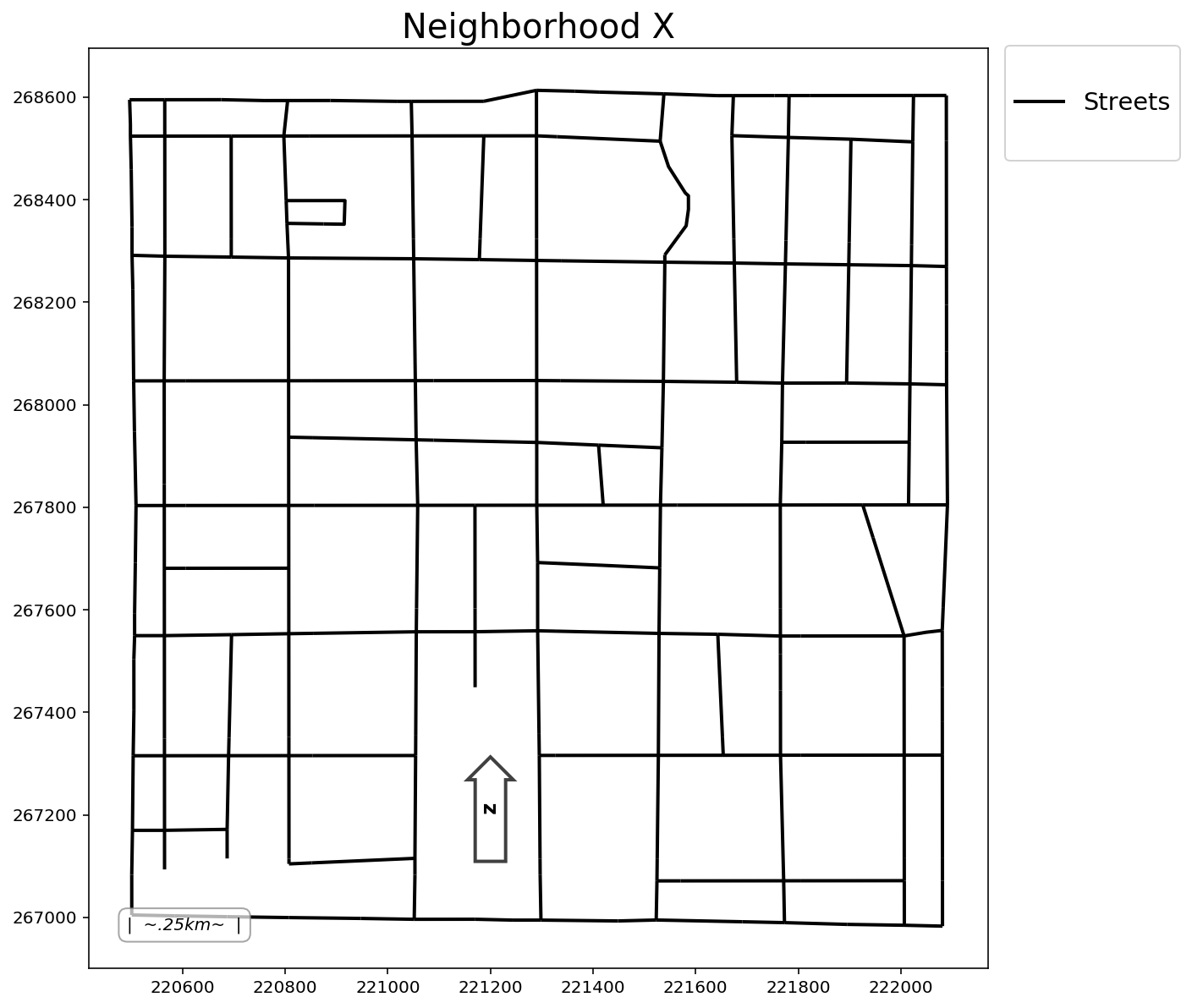### 2.2. Generate synthetic, random "houses" near the network Buffer streets buff = 50 streets_buffer = get_buffer(streets, buff=buff) streets_buffer  geometry 0 POLYGON ((222131.236 267071.937, 222131.236 26... Plot add_to_plot = {"streets": streets, "buffer": streets_buffer} plotter(plot_aux=add_to_plot, buffered=buff, title=title)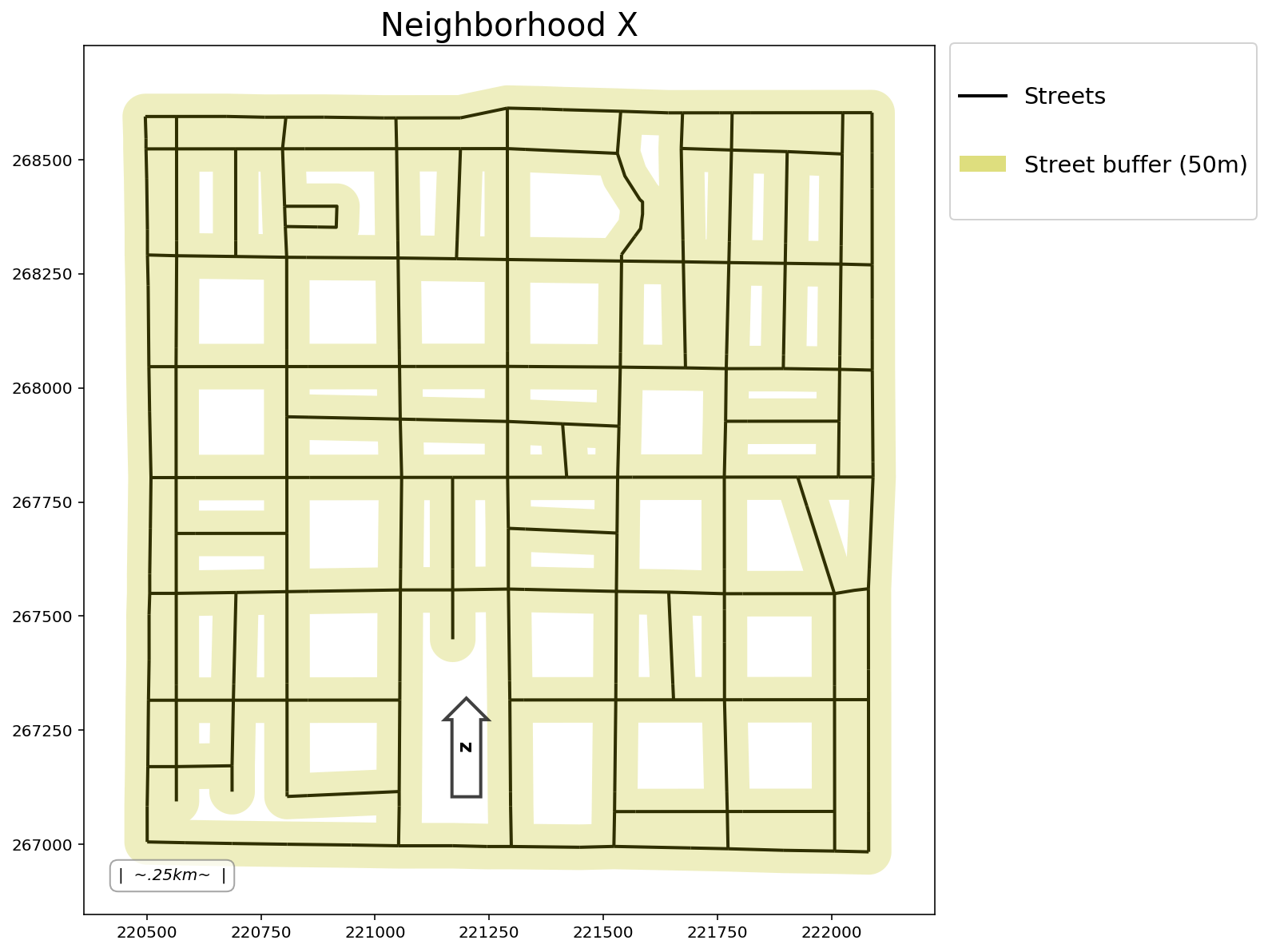Generate synthetic client points and synthetic facility points clients = simulated_geo_points( streets_buffer, needed=client_count, seed=random_seeds["client"] ) facilities = simulated_geo_points( streets_buffer, needed=facility_count, seed=random_seeds["facility"] )  Add decision variables to each dataset clients["dv"] = ["x[%s]" % c for c in range(client_count)] facilities["dv"] = ["y[%s]" % c for c in range(facility_count)]  Plot add_to_plot = { "streets": streets, "buffer": streets_buffer, "cli_tru": clients, "fac_tru": facilities, } plotter(plot_aux=add_to_plot, buffered=buff, pt1_size=60)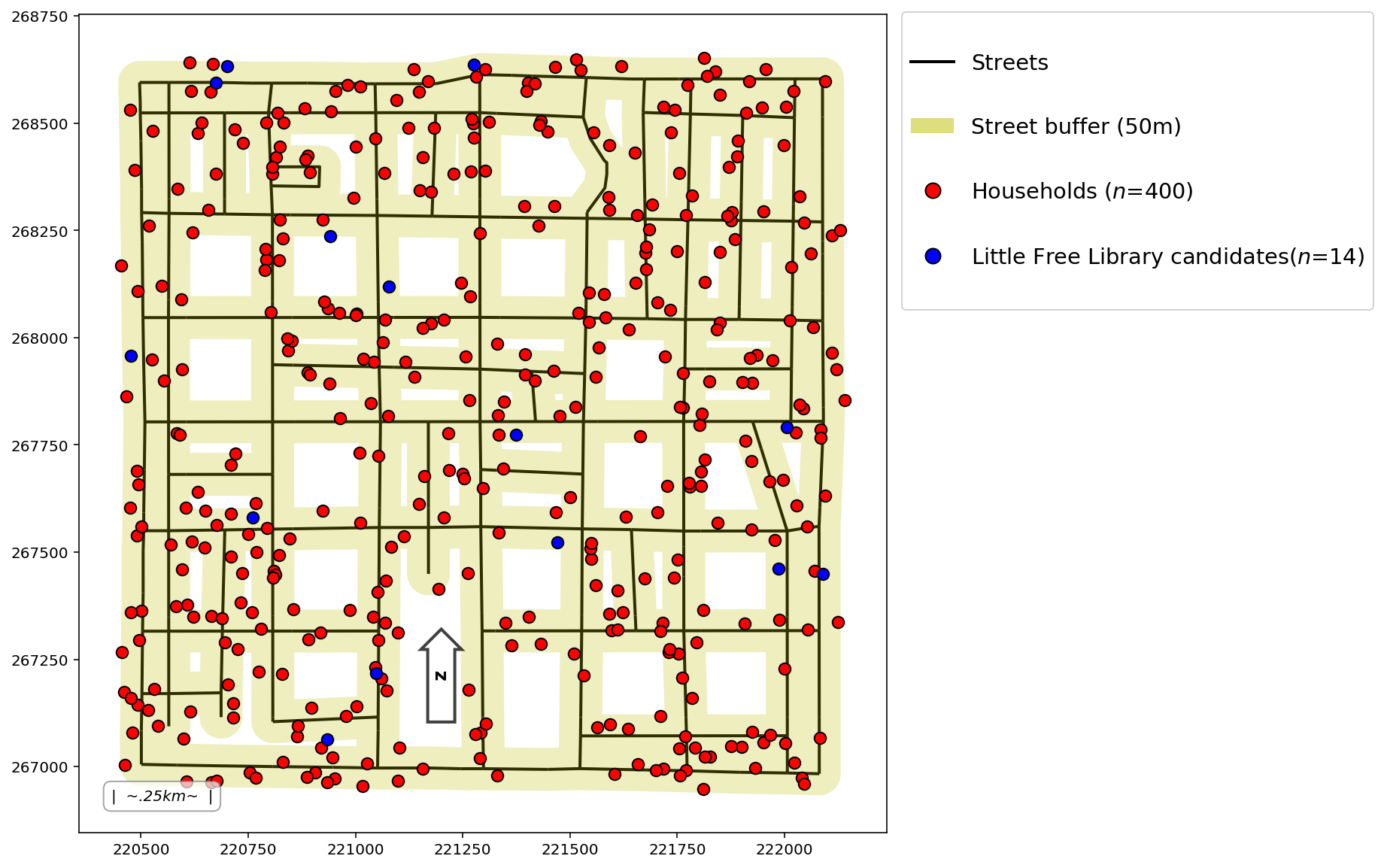### 2.3 Synthetic client demand (weights) numpy.random.seed(1991) clients["weights"] = numpy.random.randint(1, 8, (client_count, 1)) clients.head()  geometry dv weights 0 POINT (220621.368 267349.691) x 1 1 POINT (220802.618 268059.862) x 7 2 POINT (221870.232 268396.925) x 4 3 POINT (220715.450 267147.585) x 6 4 POINT (221329.906 267984.832) x 5 ### 2.4 Create a network instance ntw = spaghetti.Network(in_data=streets)  ### 2.5 Snap points to the network and fetch their dataframes ntw.snapobservations(clients, "clients", attribute=True) clients_snapped = spaghetti.element_as_gdf( ntw, pp_name="clients", snapped=True ) ntw.snapobservations(facilities, "facilities", attribute=True) facilities_snapped = spaghetti.element_as_gdf( ntw, pp_name="facilities", snapped=True )  Plot add_to_plot = { "streets": streets, "buffer": streets_buffer, "cli_tru": clients, "fac_tru": facilities, "cli_snp": clients_snapped, "fac_snp": facilities_snapped, } plotter(plot_aux=add_to_plot, buffered=buff, pt1_size=60, pt2_size=25)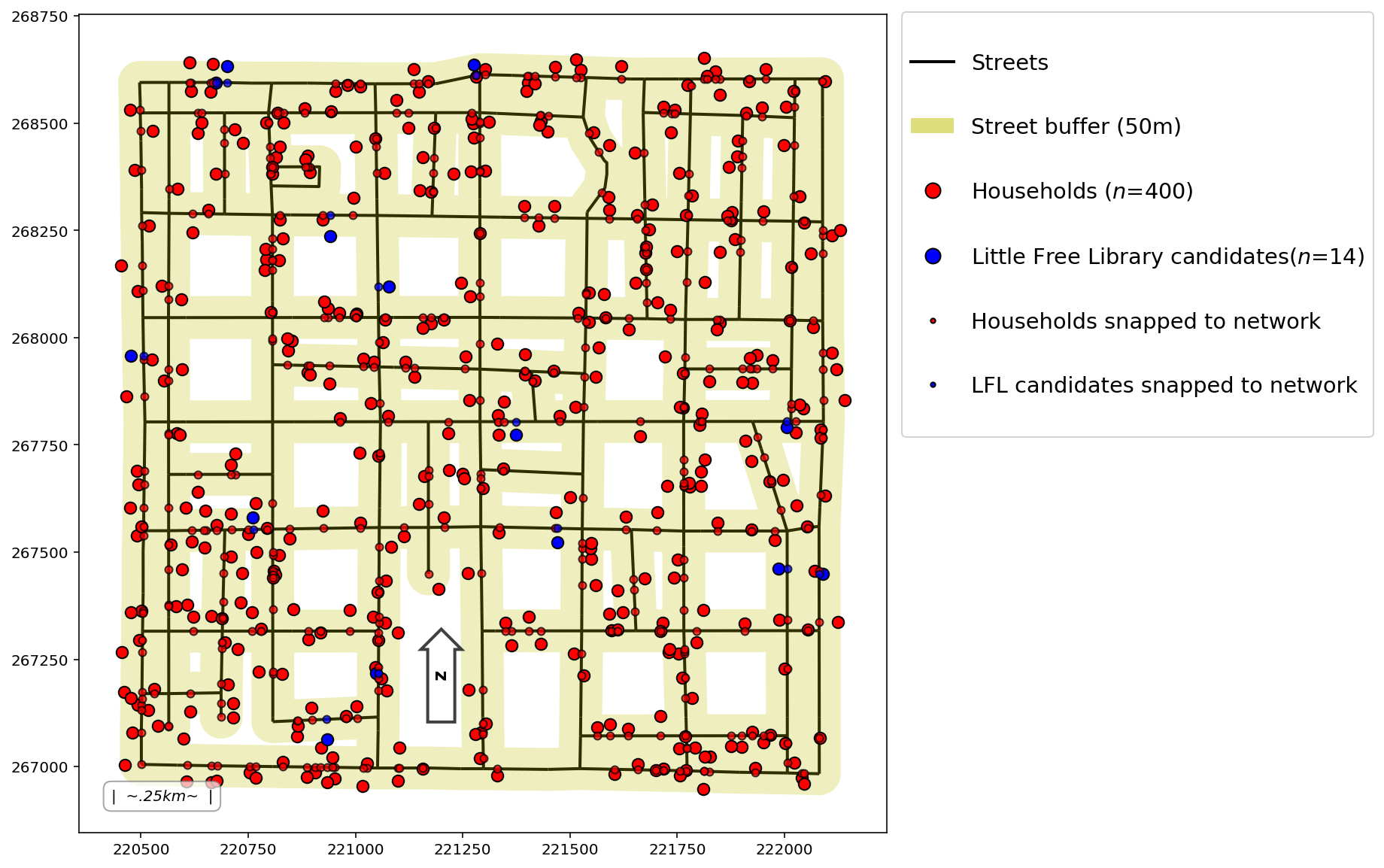### 2.6 Calculate distance matrix from all clients to all candidate facilities cost_matrix = ntw.allneighbordistances( sourcepattern=ntw.pointpatterns["clients"], destpattern=ntw.pointpatterns["facilities"], ) cost_matrix[:3, :3]  array([[1471.53181612, 1283.3690228 , 1703.75520358], [ 638.15807808, 361.05893423, 1707.02308155], [1407.3591824 , 1081.06088431, 1157.55620084]]) ### 3. Optimal Facility Location ### 3.1 Location Set Covering Problem lscp = FacilityLocationModel("lscp", cij=cost_matrix, s=max_coverage) clients, facilities = add_results(lscp, clients, facilities)  Solve Time: 0.0030333394 minutes Obj. Value: 4.0 facilities needed for total coverage within a 1000.000000 meter service radius --- 0 clients are not covered --- 400 clients are covered by 1 facility --- 185 clients are covered by 2 facilities --- 30 clients are covered by 3 facilities --- 10 clients are covered by 4 facilities  Plot aux_to_plot = {"streets": streets, "fac_tru": facilities} res_to_plot = {"cli_var": clients, "fac_var": facilities} plotter( plot_aux=aux_to_plot, plot_res=res_to_plot, pt1_size=300, pt2_size=60, model=lscp, title=title, )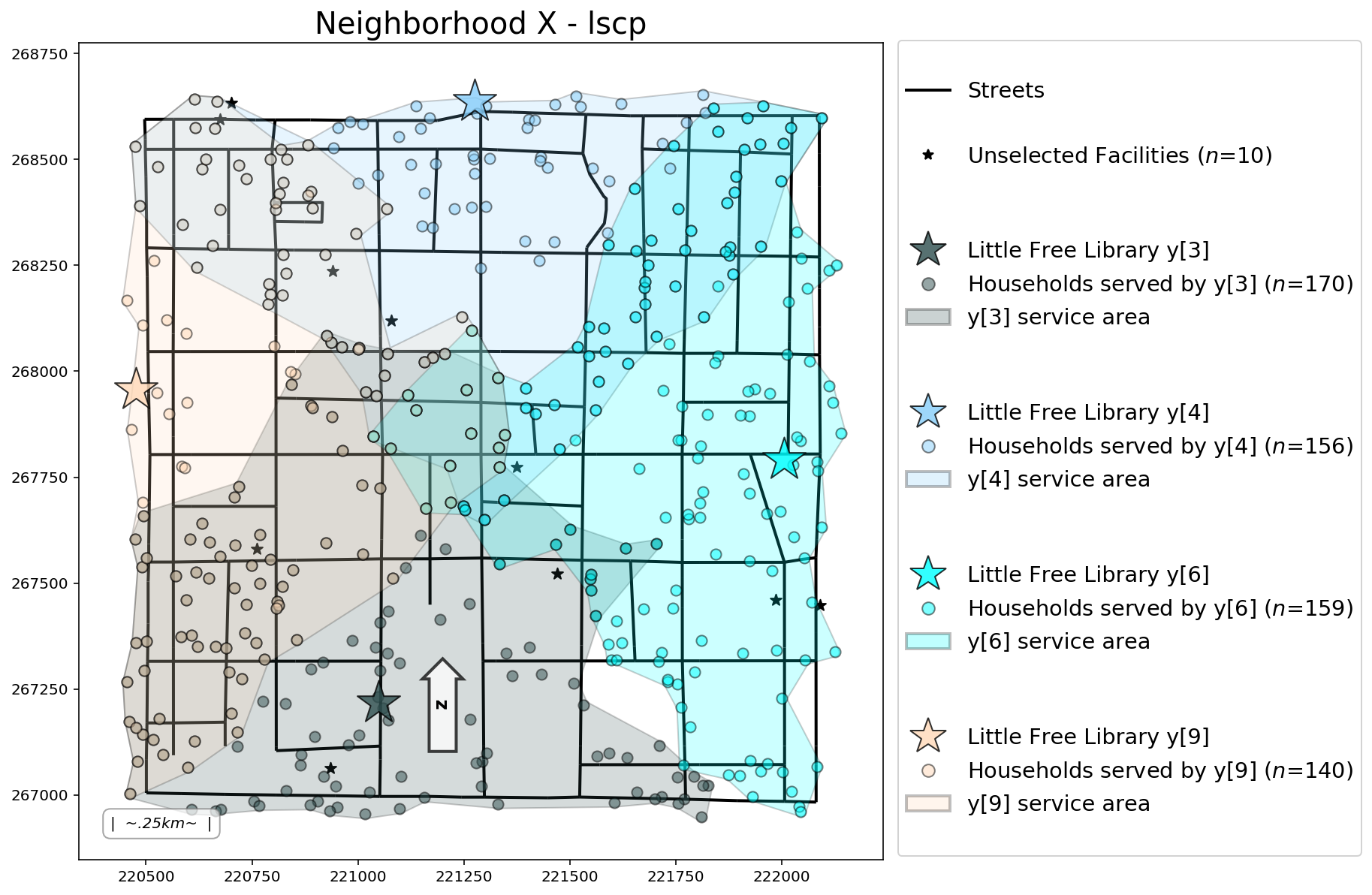### 3.2 p-median Problem pmp = FacilityLocationModel( "pmp", ai=clients["weights"], cij=cost_matrix, p=p_facilities ) clients, facilities = add_results(pmp, clients, facilities)  Solve Time: 0.014350028700000001 minutes Obj. Value: 898919.1804203619 total weighted distance with 3 selected facilities Mean weighted distance per person: 562.527647  Plot aux_to_plot = {"streets": streets, "fac_tru": facilities} res_to_plot = {"cli_var": clients, "fac_var": facilities} plotter( plot_aux=aux_to_plot, plot_res=res_to_plot, pt1_size=300, pt2_size=60, model=pmp, title=title, )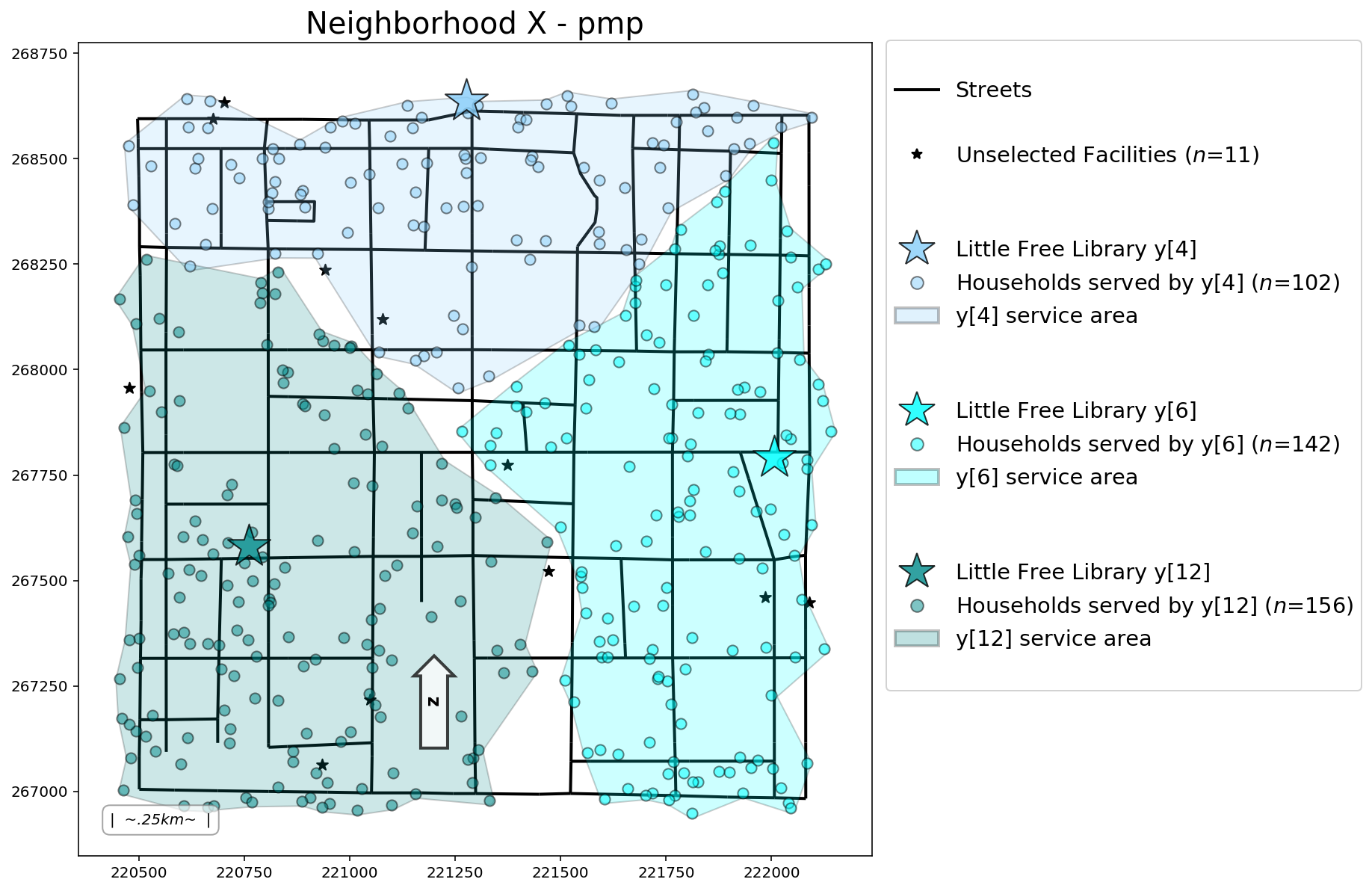### 3.3 p-center Problem pcp = FacilityLocationModel("pcp", cij=cost_matrix, p=p_facilities) clients, facilities = add_results(pcp, clients, facilities)  Solve Time: 0.06688346710000001 minutes Obj. Value: 1024.6199825236833 worst case distance with 3 selected facilities  Plot aux_to_plot = {"streets": streets, "fac_tru": facilities} res_to_plot = {"cli_var": clients, "fac_var": facilities} plotter( plot_aux=aux_to_plot, plot_res=res_to_plot, pt1_size=300, pt2_size=60, model=pcp, title=title, )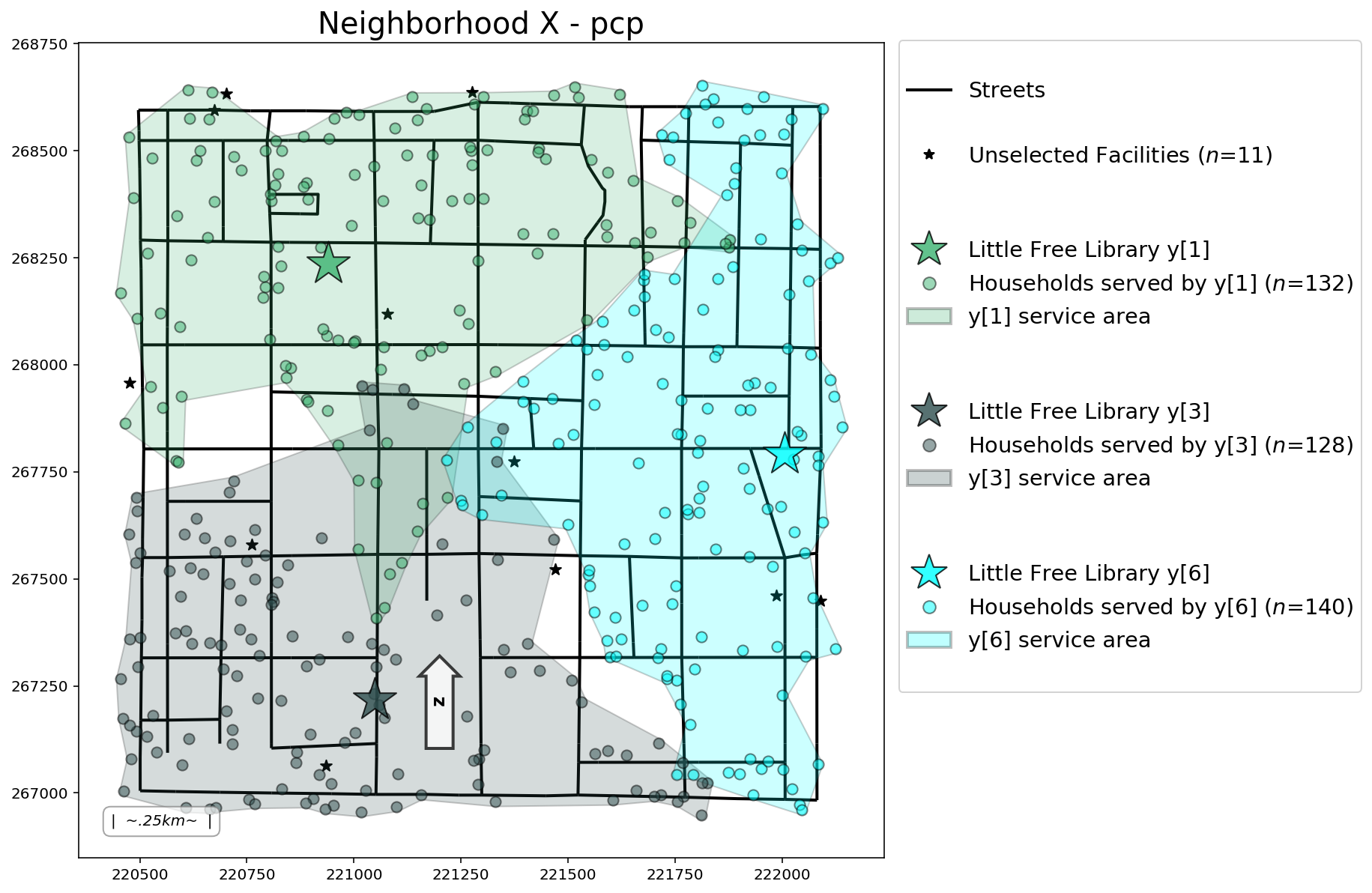### 3.4 Maximal Covering Location Problem mclp = FacilityLocationModel( "mclp", ai=clients["weights"], cij=cost_matrix, p=p_facilities, s=min_coverage ) clients, facilities = add_results(mclp, clients, facilities)  Solve Time: 0.0034500069 minutes Obj. Value: 1312.0 residents within 800.000000 meters of 3 selected facilities Percent of 1598 clients covered: 82.102628 --- 73 clients are not covered --- 327 clients are covered by 1 facility --- 17 clients are covered by 2 facilities  Plot aux_to_plot = {"streets": streets, "cli_tru": clients, "fac_tru": facilities} res_to_plot = {"cli_var": clients, "fac_var": facilities} plotter( plot_aux=aux_to_plot, plot_res=res_to_plot, pt1_size=300, pt2_size=60, model=mclp, title=title, )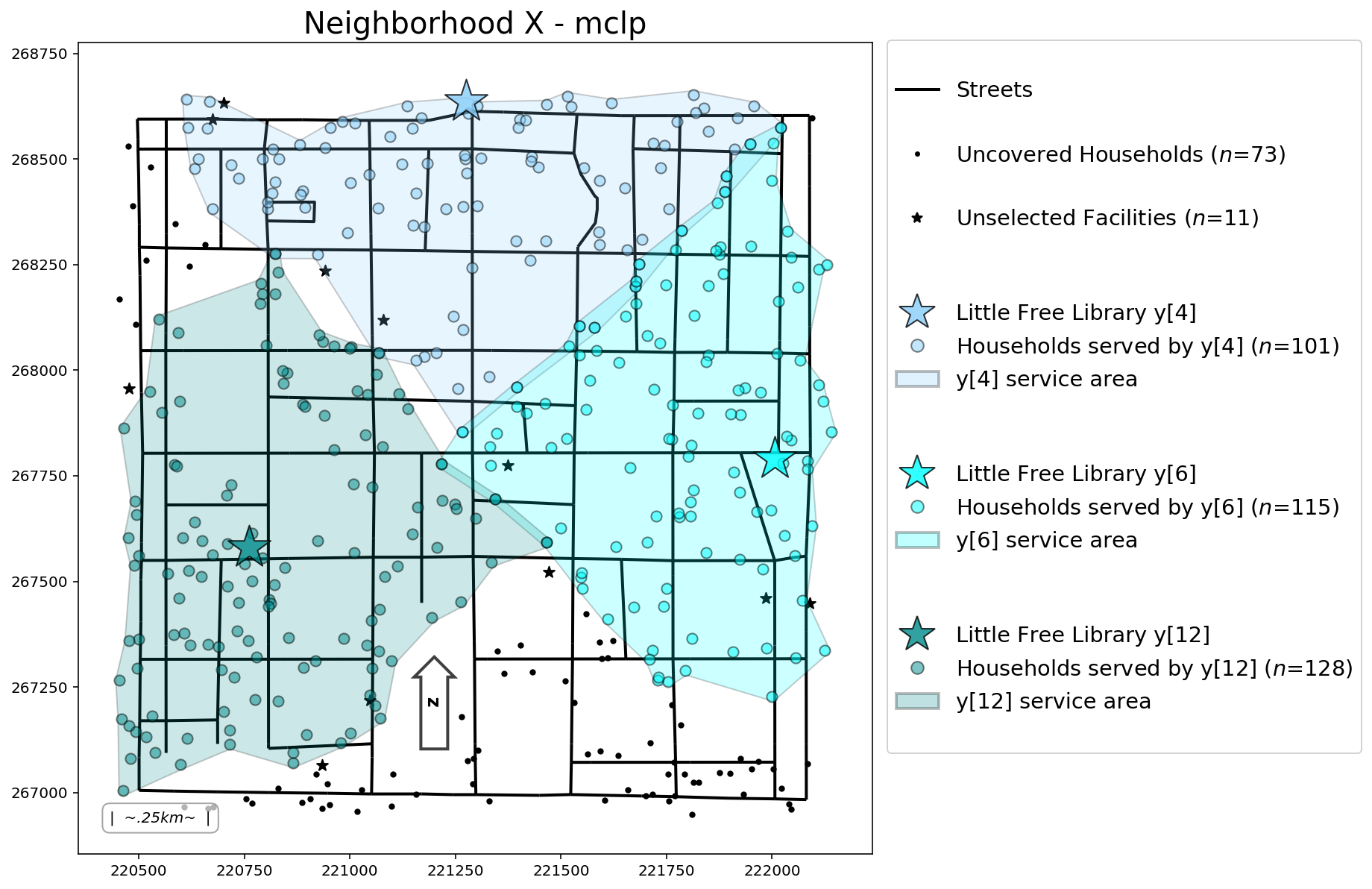### 3.5 Solved models objects models = [lscp, pmp, pcp, mclp]  ### 3.6 Distance analytics matrix$\Longrightarrow$Results may vary due to the random seed being set on local machines$\Longleftarrow$analytics_df, analytics_display = analytics_matrix(models) analytics_display  {'abs_min': 'Absolute min dist to assigned facility', 'abs_max': 'Absolute max dist to assigned facility', 'mean_means': 'Mean of mean dists per client to assigned facility', 'mean_stds': 'Mean of StD dists per client to assigned facility'} stats lscp pmp pcp mclp 0 abs_min 0.918922 0.918922 12.7455 0.918922 1 abs_max 998.526 1224.28 1024.62 799.228 2 mean_means 645.324 565.138 591.621 497.517 3 mean_stds 245.521 263.8 244.652 214.708 md(f"While it appears the {mclp.name} performs most optimally with the least distance per stastistic, we have to remember that the {mclp.name} is leaving {mclp.n_cli_uncov} clients uncovered. Therefore, it may generally give lower maximum and average travel costs due to the uncovered client travel costs being excluded.")  While it appears the mclp performs most optimally with the least distance per stastistic, we have to remember that the mclp is leaving 73 clients uncovered. Therefore, it may generally give lower maximum and average travel costs due to the uncovered client travel costs being excluded. ### 3.7 Selection matrix selection_df, selection_display = selection_matrix(models) selection_display  lscp pmp pcp mclp$\sum\%$y$\notin\notin\notin\notin$0 0 y$\notin\notin\in\notin$1 25 y$\notin\notin\notin\notin$0 0 y$\in\notin\in\notin$2 50 y$\in\in\notin\in$3 75 y$\notin\notin\notin\notin$0 0 y$\in\in\in\in$4 100 y$\notin\notin\notin\notin$0 0 y$\notin\notin\notin\notin$0 0 y$\in\notin\notin\notin$1 25 y$\notin\notin\notin\notin$0 0 y$\notin\notin\notin\notin$0 0 y$\notin\in\notin\in$2 50 y$\notin\notin\notin\notin$0 0 ### 3.8 Plot all solutions for a spatial comparision usr_warning = "The GeoDataFrame you are attempting to plot is empty" warnings.filterwarnings("ignore", message=usr_warning) # facility variable-to-times selected lookup fac2selectcount = dict(selection_df["$\sum\$"].astype(int))
aux_to_plot = {"streets": streets, "cli_tru": clients}
res_to_plot = {"cli_var": clients, "fac_var": facilities}
multi_plotter(
models,
plot_aux=aux_to_plot,
title=title,
plot_res=res_to_plot,
select=fac2selectcount,
)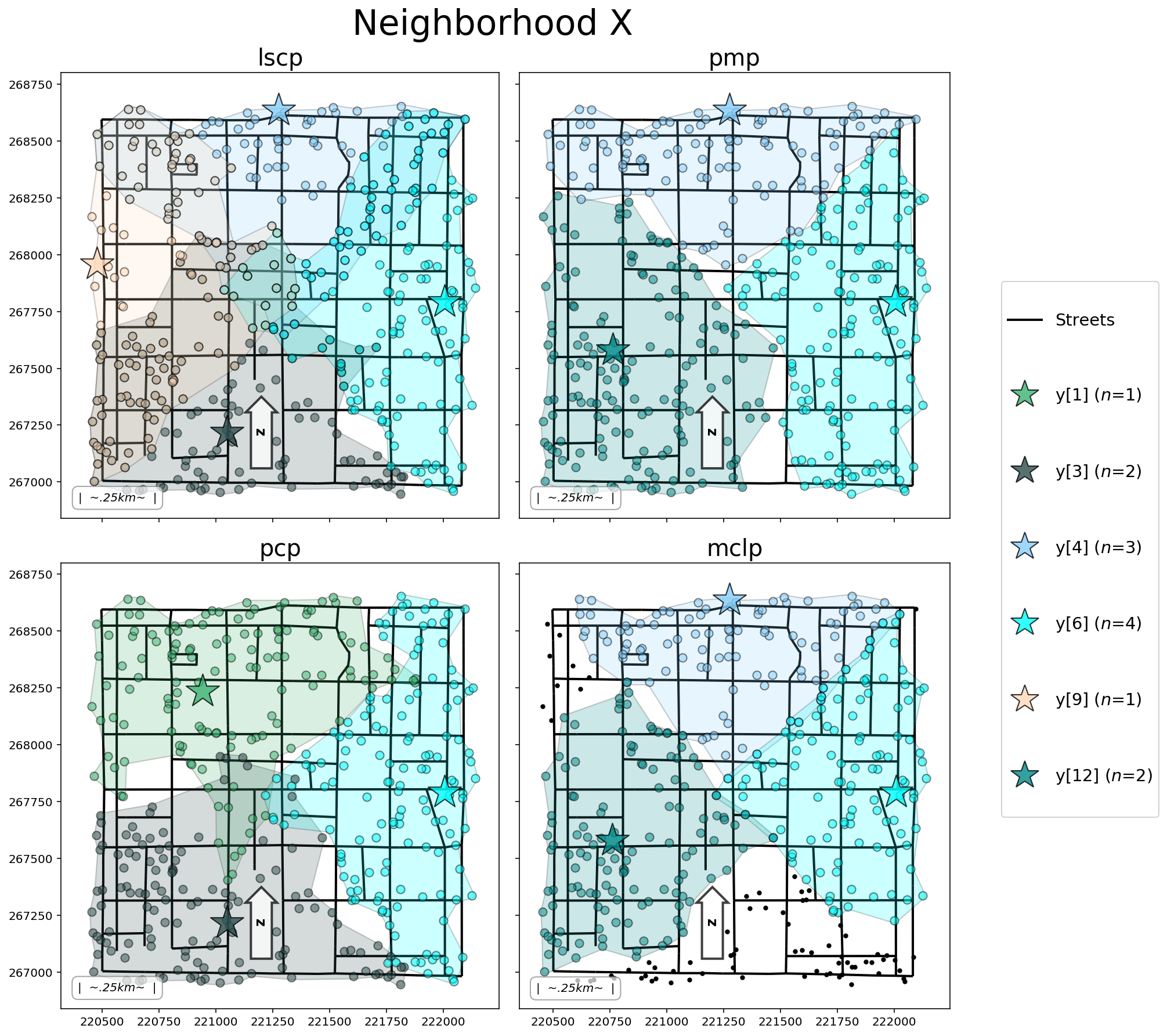### 3.9 Dr. Minimax recommendation:

v1 = pcp.name
v2 = str(list(pcp.fac2cli.keys())).translate({ord(r"'"): None})
v3 = analytics_df.loc[(analytics_df["stats"] == "abs_max"), "pcp"].squeeze()
v4 = max_coverage
md(f"* The {v1} model facility configuration: {v2} \n\
* This configuration results in an absolute maximum distance from any household to its assigned Little Free Library of {v3} meters, which is slightly more than the {v4} meter maximum distance stipulation put forth by the committee. Dr. Minimax believes this to be the most equitable and feasible solution for the residents of Neighbor X. However..., the Neighborhood X Planning Committee turned out to be corrupt and merely chose locations for the Little Free Libraries nearest to their respective houses....")

• The pcp model facility configuration: [y, y, y]
• This configuration results in an absolute maximum distance from any household to its assigned Little Free Library of 1024.6199825236833 meters, which is slightly more than the 1000.0 meter maximum distance stipulation put forth by the committee. Dr. Minimax believes this to be the most equitable and feasible solution for the residents of Neighbor X. However..., the Neighborhood X Planning Committee turned out to be corrupt and merely chose locations for the Little Free Libraries nearest to their respective houses....

The open (selected) Little Free Library Locations.

facilities[facilities["pcp_sol"] != "closed"][["dv", "geometry"]]

dv geometry
1 y POINT (220940.728 268235.675)
3 y POINT (221047.729 267218.283)
6 y POINT (222006.035 267791.360)